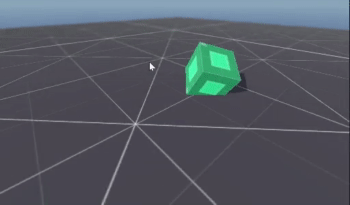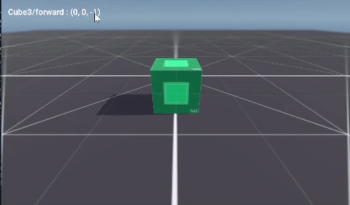# Align Movement with Camera

## Problem

When moving with WASD-style controls in 3D, it’s easy to get disoriented if the camera rotates. Whose forward counts - the player’s (ie the camera’s) or the object in the game world?

## Solution

While this situation can apply to many possible scenarios, we’ll use the Rolling Cube recipe as our example.

In the script for the cube, we have the following code for movement:

``````func _physics_process(_delta):
var forward = Vector3.FORWARD

if Input.is_action_pressed("up"):
roll(forward)
if Input.is_action_pressed("down"):
roll(-forward)
if Input.is_action_pressed("right"):
roll(forward.cross(Vector3.UP))
if Input.is_action_pressed("left"):
roll(-forward.cross(Vector3.UP))
``````

As you can see, this uses global direction vectors, so if the camera rotates, “up” will no longer appear to be moving forward in the camera view. If we rotate the camera 180°, everything will be reversed!

We could just change to use the camera’s forward vector:

``````var forward = -camera.transform.basis.z.normalized()
``````

For some setups this might be fine. However, it really doesn’t work for the cube:The cube needs to only move in the 4 cardinal directions. This means we need to take the camera’s forward vector and find which of the axes (+X, -X, +Z, or -Z) it’s closest to.

We can find this using the `Vector3.max_axis()` function. This returns which of the vector’s components is the largest. Since they can be positive or negative, we’ll use `abs()` first.

Once we have the axis with the largest magnitude, we can set our `forward` vector to match:

``````func _physics_process(_delta):
var forward = Vector3.FORWARD
if camera:
forward = Vector3.ZERO
var cam_forward = -camera.transform.basis.z.normalized()
var cam_axis = cam_forward.abs().max_axis()
forward[cam_axis] = sign(cam_forward[cam_axis])

if Input.is_action_pressed("up"):
roll(forward)
if Input.is_action_pressed("down"):
roll(-forward)
if Input.is_action_pressed("right"):
roll(forward.cross(Vector3.UP))
if Input.is_action_pressed("left"):
roll(-forward.cross(Vector3.UP))
``````

In this clip, I’m only pressing “w” to move: